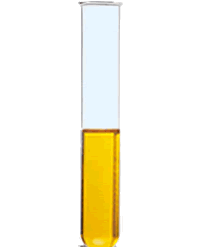# What is the colour change when aqueous iron(II) ions are treated with an acidified solution of dichromate(VI) ?

Apr 13, 2016

The colour change is from pale blue/green to yellow/brown.

#### Explanation:

Dichromate(VI) is a powerful oxidising agent and takes in electrons according to the 1/2 equation:

$C {r}_{2} {O}_{7 \left(a q\right)}^{2 -} + 14 {H}_{\left(a q\right)}^{+} + 6 e \rightarrow 2 C {r}_{\left(a q\right)}^{3 +} + 7 {H}_{2} {O}_{\left(l\right)} \text{ } \textcolor{red}{\left(1\right)}$

The iron(II) ions give up their electrons according to:

$F {e}_{\left(a q\right)}^{2 +} \rightarrow F {e}_{\left(a q\right)}^{3 +} + e \text{ } \textcolor{red}{\left(2\right)}$

To get the electrons to balance you can see that we need to multiply $\textcolor{red}{\left(2\right)}$ by 6 then add this to equation $\textcolor{red}{\left(1\right)} \Rightarrow$

Cr_2O_(7(aq))^(2-)+14H_((aq))^(+)+cancel(6e)+6Fe_((aqa))^(2+)rarr6Fe_((aq))^(3+)+cancel(6e)+2Cr_((aq))^(3+)+7H_2O_((l))"

Iron(II) solutions contain the hexaaquoiron(II) ion ${\left[F e {\left({H}_{2} O\right)}_{6}\right]}^{2 +}$ which is a pale blue/green colour.

In the solid, hydrated state they look like this:In aqueous conditions a solution of iron(III) contains the hexaaaquoiron(III) ion [Fe(H_2O)_6]^(3+)]. The solution looks a yellow/brown colour:The colour is actually yellow due to the hydrolysis of the ion in water:

${\left[F e {\left({H}_{2} O\right)}_{6}\right]}^{3 +} + {H}_{2} {O}_{\left(l\right)} r i g h t \le f t h a r p \infty n s {\left[F e {\left({H}_{2} O\right)}_{5} \left(O H\right)\right]}^{2 +} + {H}_{3} {O}^{+}$

It is the ${\left[F e {\left({H}_{2} O\right)}_{5} \left(O H\right)\right]}^{2 +}$ that gives the yellow/brown colour.

Pure iron(III) ions are actually pale violet. This is what they look like in the solid state:However, these colours will be masked when you carry out the reaction.

Potassium chromate(VI) is orange in colour and that's what you see before the reaction. These are converted to green $C {r}^{3 +}$ ions so you will see a mixture of these and the iron(III) species after the reaction.